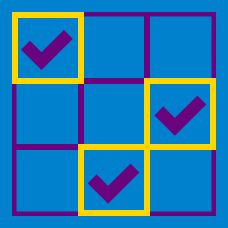Logic

# Grid Puzzles: Level 3 Challenges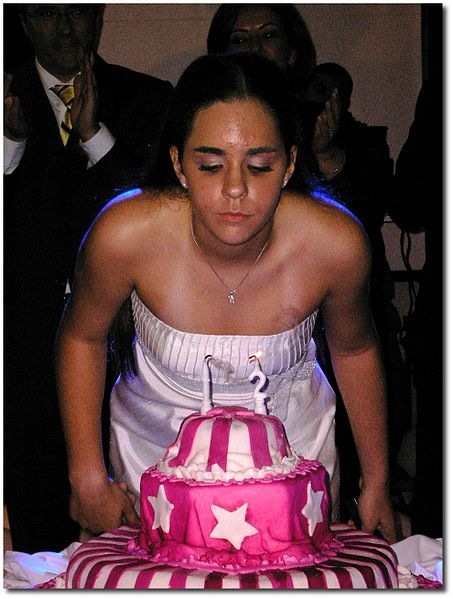Albert and Bernard just became friends with Cheryl, and they want to know when her birthday is. Cheryl gives them a list of 10 possible dates:

 May 15 May 16 May 19 June 17 June 18 July 14 July 16 August 14 August 15 August 17

Cheryl then tells Albert and Bernard separately the month and the day of her birthday respectively.

Albert: I don't know when Cheryl's birthday is, but I know that Bernard does not know too.
Bernard: At first I don't know when Cheryl's birthday is, but I know now.
Albert: Then I also know when Cheryl's birthday is.

So, when is Cheryl's birthday?

###### Image credit: Wikipedia Tatiana SapateiroDuring a meeting from their school mathematics club, Andy, Becky, Chloe and Danny discover that their favourite number (a positive integer) is the same. So, each of the four friends makes three statements about the number, at least one of which is true and at least one of which is false.

$\begin{matrix} \textbf{Andy}: & \textit{(A1)} & \text{The number is less than 12}\\ & \textit{(A2)} & \text{7 does not divide the number}\\ & \textit{(A3)} & \text{5 times the number is less than 70}\\ \textbf{Becky}: & \textit{(B1)} & \text{12 times the number is greater than 1000}\\ & \textit{(B2)} & \text{10 divides the number}\\ & \textit{(B3)} & \text{The number is greater than 100}\\ \textbf{Chloe} : & \textit{(C1)} & \text{4 divides the number}\\ & \textit{(C2)} & \text{11 times the number is less than 1000}\\ & \textit{(C3)} & \text{9 divides the number}\\ \textbf{Danny} : & \textit{(D1)} & \text{The number is less than 20}\\ & \textit{(D2)} & \text{The number is a prime number}\\ & \textit{(D3)} & \text{7 divides the number} \end{matrix}$

What is their favorite number?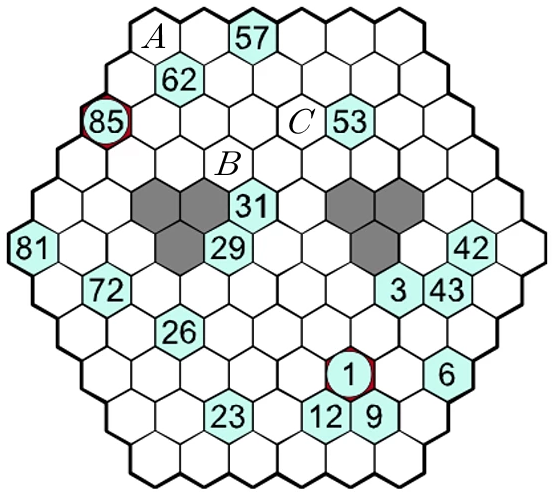The picture above shows a Hidato puzzle. The aim of the puzzle is to fill each white/light blue cell with an integer between 1 and 85 (inclusive) so that each integer appears exactly once and consecutive integers appear in adjacent cells.

Let the number that takes place of the cell marked $A$ be denoted $A$, and so forth.

What is the value of $A+B+C$?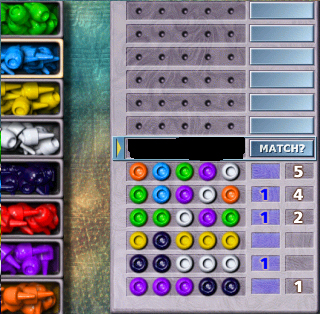In the game Mastermind, I have to guess some permutation of five colors out of eight (being a permutation, all colors are different). I can submit a guess, and I receive a reply in the form of two numbers:

• The blue number tells the number of colors in my guess that exist in the secret pattern, which are also correct in position.
• The white number tells the number of colors in my guess that exist in the secret pattern, but which are incorrect in position.

When where there should be a number, it is empty instead, the number is assumed to be zero.

What is the secret pattern? Enter your answer in order from left to right, translating the color to a digit code according to this table:

 Color Green Blue Yellow White Code 1 2 3 4 Color Black Red Purple Orange Code 5 6 7 8

As an example, if the answer is orange-blue-yellow-white-black, enter $82345$.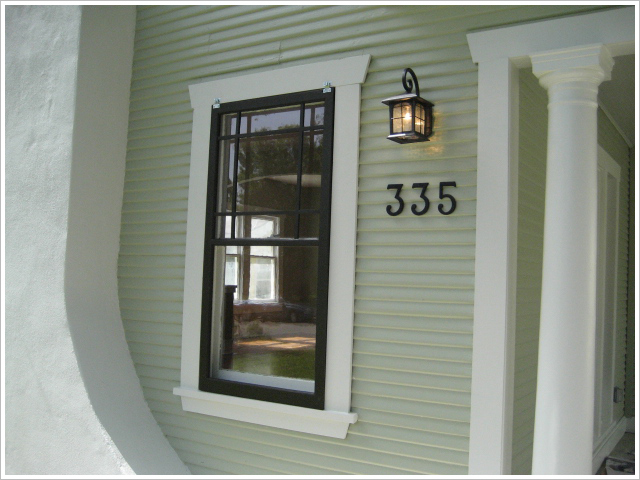A maths professor asked one of his students to visit his house regarding some work. The student asked the professor his house number. The professor replied in a strange way:

1. If my house number is a multiple of 3, then it is a number from 50 to 59.

2. If my house number is not a multiple of 4, then it is a number from 60 to 69.

3. If my house number is not a multiple of 6, then it is a number from 70 to 79.

Can you determine the professor's house number?

×